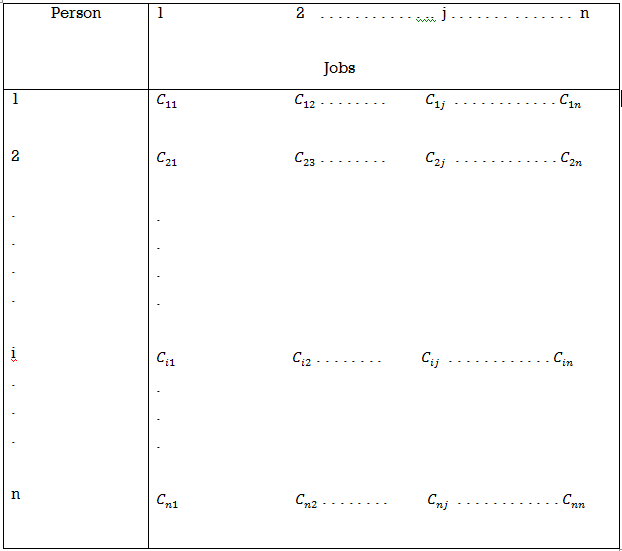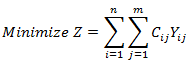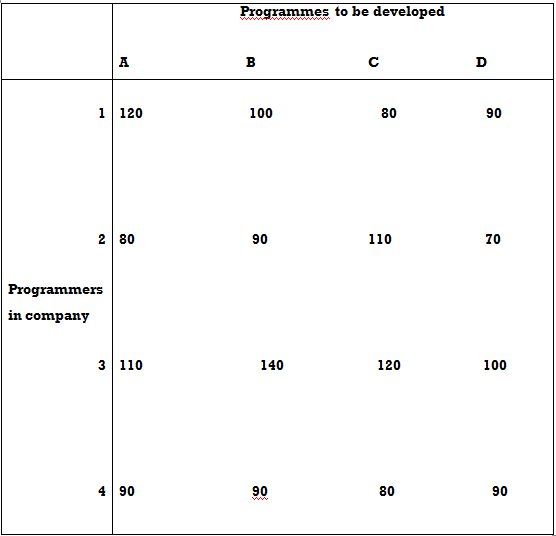Goseeko blog# What is an assignment problem?

## Overview(assignment problem)

When one task is to assign to one person in such a way that the total person hours are to be minimize, then this kind of problem is a assignment problem.

Assignment problems are the special case of transportation problems. In assignment problems the number of sources and destinations are same.

## Formulation of cost matrix

Let there are n persons and n jobs and the assignment of jobs to do on a one-to-one basis. We can state this assignment problem in the form of an n×n matrix of real numbers which we call it cost matrix.Here Cij is the amount of time that by i’th person take to complete jth job.

Suppose Yij denotes the jth job assigned to the ith person.

Then the mathematical representation of assignment problem will be as follows

Here Cij is the amount of time that i’th person take to complete jth job.

Suppose Yij denotes the jth job assigned to the ith person.

Then the mathematical representation of assignment problem will be as followsWhere i = 1,2,…,n and j = 1,2,..,m

Subject to

## Hungarian method

To solve any assignment problem easily, we use Hungarian method.

Step by step procedure

Consider a minimization type objective function. Resolving this allocation issue involves the subsequent steps:

Step-1

Find the littlest cost element in each row of the required cost table, starting with the primary row. Here, this smallest element is subtracted from each element therein row. Therefore, get a minimum of one zero in each row of this new table.

Step-2

After creating the table (as in step 1), get the columns of the table. Start with the primary column and find rock bottom cost think about each column. Then subtract this smallest element from each element therein column. After performing steps 1 and a couple of, you’ll see a minimum of one zero in each column of the reduced cost table.

Step-3

The table is now allocate within the following way:

(I) Rows are continuously inspected until a row with just one zero is found. This assignment to one zero is formed by placing a square □ around it, with all other zeros being strikethrough (x) within the corresponding column. this is often because they’re not used for other assignments during this column. The steps are performed for every row.

(Ii) Step 3 (i) am performed on the column as follows: -Columns are continuously inspected until a column with just one zero is found. Now, by placing a square around it, this single zero is assigned, and at an equivalent time all other zeros within the corresponding row are erased (x) A step is performed on each column. I will.

(Iii) Steps 3, (i), and three (ii) are repeated until all zeros are marked or strikethrough is drawn. Here, if the amount of zeros marked or the amount of allocations made is adequate to the amount of rows or columns, the simplest solution is achieved. There’s exactly one assignment for every column or column, with none assignment. During this case, attend step 4.

4. At this stage, draw the minimum number of lines (horizontal and vertical) needed to hide all the zeros within the matrix obtained in step 3. Use the subsequent procedure.

(i) Put a check on all unassigned lines.

(ii) Now mark of these columns that have zeros within the graduated rows (iii) Now check all rows that haven’t yet been marked and are assigned to the marked columns.

(iv) All steps, namely (4 (i), 4 (ii), 4 (iii), are repeated until no row or column are often marked.

(V) Draw a line through all unmarked rows and marked columns. Also note that in an n x n matrix, lines but “n” always cover all zeros if there’s no solution between them.

5. In step 4, the amount of lines is n or the number of lines, it’s the best solution. If not, go to step 6.

6. Select the smallest element from all uncovered elements. Here, this element is to subtract from all uncovered elements and add to the element at the intersection of the two lines. This is the new allocation matrix.

7. Repeat step (3) until the number of allocations is equal to the number of rows or columns.

## Solved example of assignment problem

Example: A software company has four expert programmers and needs to develop four application programmes. The manager of the company, estimates the computer time (in minutes) required by the respective experts to develop the application programmes as follows:Find the assignment pattern that minimises the time required to develop the application programmes.

Sol.

Let us subtract the minimum element of each row from every element of that row. Note that the minimum element in the first row is 80. So 80 is to subtract from every element of the first row, i.e., from 120, 100, 80 and 90, respectively. As a result, the elements of the first row of the resulting matrix would be 40, 20, 0, 10, respectively. Similarly, we obtain the elements of the other rows of the resulting matrix. We obtain,

the resulting matrix. We obtain,

Now subtract the minimum element of each column from every element of that column in the resulting matrix. The minimum element in the first column is 10. So 10 to subtract from every element of the first column, i.e., from 40, 10, 10, and 10, respectively. As a result, the elements of the first column of the resulting matrix are 30, 0, 0, 0, respectively. Similarly, we obtain the elements of the other columns of the resulting matrix. We obtain,

Now, starting from first row onward, we draw a rectangle around the 0 in each row having a single zero and cross all other zeroes in the corresponding column. Here, in the very first row we find a single zero. So, we draw a rectangle around it and cross all other zeroes in the corresponding column.

We obtain

In the second, third and fourth row, there is no single zero. Hence, we move column-wise. In the second column, we have a single zero. Hence, we draw a rectangle around it and cross all other zeroes in the corresponding row.

We obtain

In the matrix above, there is no row or column, which has a single zero.

Therefore, we first move row-wise to locate the row having more than one zero. The second row has two zeroes. So, we draw a rectangle arbitrarily around one of these zeroes and cross the other one. Let us draw a rectangle around the zero in the cell (2, A) and cross the zero in the cell (2, D). We cross out the other zeroes in the first column. Note that we could just as well have selected the zero in the cell (2, D), drawn a rectangle around it and crossed all other zeroes. This would have led to an alternative solution.

In this way, only one zero in every row and column around which a rectangle has been drawn. This means that we have assigned only one operation to one operator. Thus, we obtain the optimum solution as follows:

Here the assignment of jobs should be made on the basis of the cells corresponding to the zeroes around which rectangles have been drawn.

Therefore, the optimum solution for this problem is:

1 = C, 2 = A, 3 = D, 4 = B

This means that programmer 1 is assigned programme C, programmer 2 is assigned programme A, and so on. The minimum time taken in developing the programmes is

= 80 + 80 + 100 + 90 = 350 min.

Interested in learning about similar topics? Here are a few hand-picked blogs for you!